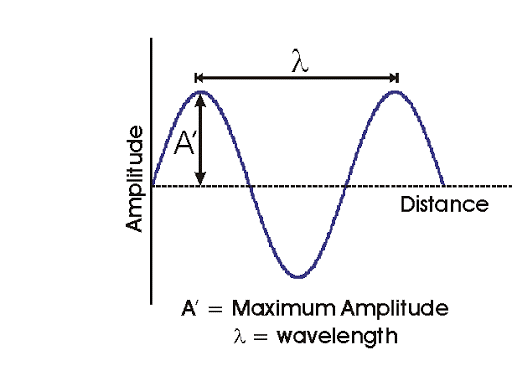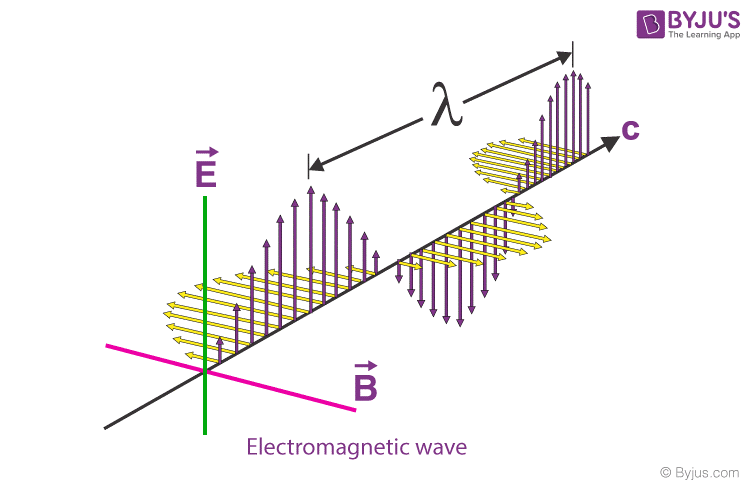# Waves

## Definition:

A wave is a perturbation in a medium that carries energy without a net particle movement. It can be in the form of elastic deformation, pressure variance, electric or magnetic strength, electrical power, or temperature.

## Introduction of Waves• Control transitions.

ntent -->
• Normally a regular movement is involved.

• The medium or particles (mechanical wave) cannot pass net. That is not the result.

• There are some fundamental wave descriptors. The gap for a similar component of the wave is the wavelength.

• Amplitude is the neutral position ‘s full displacement. This indicates the wave ‘s control. Greater amplitude is more energy-consuming. Displacement is the location at a certain point in the medium as the wave moves through. The wave amplitude is the highest displacement.

• Frequency (ƒ) is the number of repetitions per second in Hz, s -1 is the time to travel around a point of a wavelength. T = f-1.

• The wave speed (v) is the speed at which a single segment of the wave moves. A light wave ‘s amplitude is c.

## Types of Waves:

the types of waves are given below.

## Transverse Waves

Waves in which the medium moves at right angles to the direction of the wave.

Examples of transverse waves:

• Water waves (ripples of gravity waves, not sound through water)
• Light waves
• S-wave earthquake waves
• Stringed instruments
• Torsion wave
• The high point of a transverse wave is a crest. The low part is a trough.

## Longitudinal Wave:

A longitudinal wave has the movement of the particles in the medium in the same dimension as the direction of movement of the wave.

Examples of longitudinal waves:

• Sound waves
• P-type earthquake waves
• Compression wave

## Parts of longitudinal waves:

Compression: where the particles are close together.

Rarefaction: where the particles are spread apart.

## Electromagnetic Waves:

Radio signals, light rays, x-rays, and cosmic rays.

## Mechanical waves:

A wave which needs a medium in order to propagate itself. Sound waves, waves in a Slinky, and water waves are all examples of this.

## Matter Waves:

Any moving object can be described as a wave When a stone is dropped into a pond, the water is disturbed from its equilibrium positions as the wave passes; it returns to its equilibrium position after the wave has passed.

## Electromagnetic Waves:These waves are the disturbance that does not need any object medium for propagation and can easily travel through the vacuum. They are produced due to various magnetic and electric fields. The periodic changes that take place in magnetic electric fields and therefore known as Electromagnetic Wave

It is the total distance covered by the wave in a given time period. The formula for wave speed is given as,

Wave Speed = Distance Covered/Time taken

## Properties of Waves

The prime properties of waves are as follows:

### Amplitude

Wave is an energy transport phenomenon. Amplitude is the height of the wave, usually measured in meters. It is directly related to the amount of energy carried by a wave.

### Wavelength

The distance between identical points in the adjacent cycles of crests of a wave is called a wavelength. It is also measured in meters.

### Period

The period of a wave is the time for a particle on a medium to make one complete vibrational cycle. As the period is time, hence is measured in units of time such as seconds or minutes.

## Frequency

The frequency of a wave is the number of waves passing a point in a certain time. The unit of frequency is hertz (Hz) which is equal to one wave per second.

The period is the reciprocal of the frequency and vice versa.

Period=1Frequency

OR

Frequency=1Period

## Speed

The speed of an object means how fast an object moves and is usually expressed as the distance travelled per time of travel. The speed of a wave refers to the distance travelled by a given point on the wave (crest) in a given interval of time. That is –

Speed=Distance Time

Speed of a wave is thus measured in meter/second i.e. m/s.

#### Related Posts